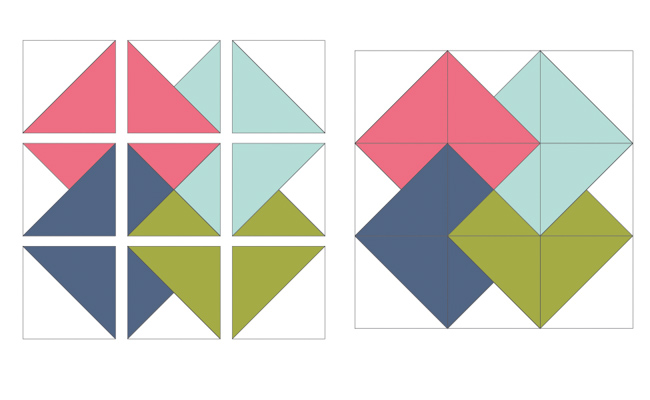# How to Sew a Perfect Quarter-Square Triangle

Quarter-square triangles are used in dozens of quilt blocks, and making them is a great skill for quilters of all levels of expertise. Read on to learn the ins and outs of creating your own!

## What is a quarter-square triangle?

A quarter-square triangle is a square fabric unit made up of four right triangles.The quarter-square triangle unit is used in many different patchwork quilt patterns, and there are several ways to make a quarter-square triangle unit.

## How to make a quarter-square triangle

The simplest way to make quarter-square triangles is starting with half-square triangles, and that’s what I’ll show you in this tutorial.

### Step 1: Calculate the size

Great news: There’s a very simple formula you can use to figure out what size fabric pieces you need to start with to make a quarter-square triangle of any size. Here’s the formula:

#### Finished block size + 1¼”

Once you know the starting size for your fabric, gather at least two contrasting fabrics of that size. (I’m using Lily & Loom Dreamfield for this tutorial.)### Step 2: Make half-square triangles.

1. Start with two contrasting squares 1¼” larger than the finished size you need.
2. Place squares right sides together.
3. Mark a line, corner to corner, on the wrong side of the fabric.
4. Sew ¼” away from the drawn line on each side.
5. Use a rotary cutter and ruler to cut on the drawn line.
6. Press seams toward the darker fabric.### Step 3: Turn the half-square triangles into quarter-square triangles

Once the half-square triangles are made, it’s time to make them into quarter-square triangles. Using two identical half-square triangles will result in two identical quarter-square triangle units. These symmetrical units are often referred to as hourglass blocks.1. Place the two half-square triangle units right sides together in the opposite direction (so the same fabrics do not stack atop each other). The seams should nest.2. Draw a line on the diagonal that’s perpendicular to the seam line. The line will run across the seams. Pin in place to prevent seams from shifting.Sew ¼” on each side of the drawn line. Then cut on the drawn line to create two pieces.Press units open.

## More variations of quarter-square triangles

A few simple swaps can completely change the look of your quarter-square triangles! Here are a few ways to change things up.

### 1. Mix-and-matchStart with two different half-square triangle units (instead of two made with the same fabric) to make a scrappy quarter-square triangle unit. Follow the same directions as above to make these quarter-square triangle units.

### 2. Half-square plus quarter-square

Swap out one of the half-square triangle units for a plain square for another variation of a quarter-square triangle unit.

Follow the same directions as above to make these quarter-square triangle units.  This variation results in two mirrored quarter-square triangle units.## Make any size quarter-square triangle

Below is a cheat sheet to construct any size quarter-square triangle unit. Keep in mind that you will end up with two quarter-square triangle units.

One pair of half-square triangles equals one pair of quarter-square triangles.## Can’t get your units just right? Here’s what might be going wrong…

### If you have wonky units

The quarter-square triangle unit has bias edges, which stretch and become wonky and distorted easily. Don’t be afraid to use starch to help the fabrics hold their shape. Using pins to sew the units will also help them keep their shape better.

### If your units are too small

The formula we use requires precise ¼” seam allowances, so double check that you’re sewing those seams accurately.

Pressing the units well will help them keep their size. Seams pressed to the side can also add bulk and shrink the units. If the units are coming out too small, try pressing the seams open. Pin well to line up seams when pressing seams open.

Another option is to start with slightly larger squares than the formula calls for. Then, trim units to the correct size once they’re all sewn.

## Ready to give it a go? Use your quarter-square triangles in these blocks.

### 1. Hourglass blockThe hourglass block is used as a stand-alone block in many quilts or as a unit of a quilt block. Two identical half-square triangle units make up this block.

###The card trick block is made up of four quarter-square triangle units, four half-square triangle units and one mixed quarter-square triangle unit.

### 3. Double pinwheel blockThe double pinwheel block is made up of four identical quarter-square triangle units.  Simply rotate each quarter-square triangle unit to make the double pinwheel block.

### 4. Ohio Star BlockThis classic quilt block is made up of four identical hourglass blocks, plus five solid squares.